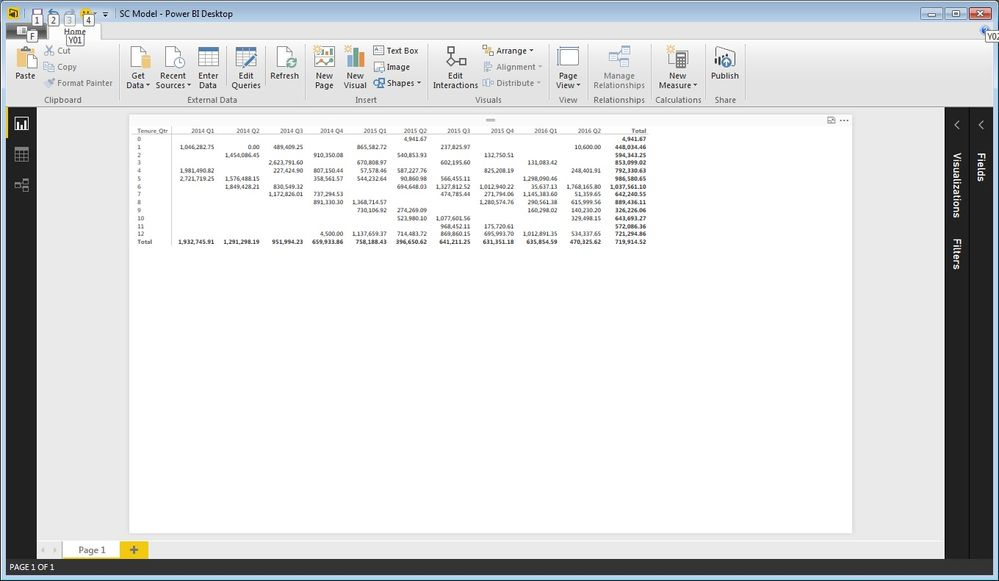cancel
Showing results for
Did you mean:Helper I

## Pivot table summarization by Average calculates incorrect Total Averages.

This pivot is summarized by Average. The body of the pivot Averages correctly. The totals are whack. (Technical term).2014 Q1 Average should be 1,916,497.61

1 ACCEPTED SOLUTIONResident Rockstar

Blanks are ignored in DAX. If 0 is represented in your data by a null / blank then that row will not be considered in the average in the numerator or denominator.

11 REPLIES 11Anonymous
Not applicable

What should the averages be?  Do you want to average all values that contain 0 for that month?Helper I

Using "2014 Q1" as an example.

There are 3 values that total to \$5,749,493.

(\$5,749,493 / 3) = \$1,916,497.6

FYI, this is how Excel computes Average.

Jeff KerykResident Rockstar

If using the default average aggregation, the resulting measure is equivalent to defining a measure as follows:

```// DAX
// Measure

Average =
AVERAGE( 'Table'[Field] )```

This will add up every value in the field and divide by the count of values. If you have granular data, we should not expect the grand total average be the same as the sum of the displayed subtotals divided by the count.

The AVERAGE() function is equivalent to the following:

```// DAX
// Measure

Average2 =
SUM( 'Table'[Field] ) / COUNT( 'Table'[Field] )```

So, if you have more entries in your data model table than display fields in the matrix, then your grand total will not be simply the sum of displayed values divided by the count.

Can you share your model structure and / or some sample data recreating this issue with us?Super User

@jeffreykeryk - To provide a concrete example of what @greggyb is saying, consider the following simple math scenario:

1

2

3

12

The average of this is 4.5 (18/4)

4

5

6

The average of this is 5 (15/3)

9.5 / 2 = 4.75 Average

However, if you take the average of all of the numbers 1+2+3+12+4+5+6 = 33 / 7 = 4.7142857...

Become an expert!: Enterprise DNA
External Tools: MSHGQM
Latest book!:
Mastering Power BI 2nd EditionDAX is easy, CALCULATE makes DAX hard...Helper ISuper User

If you add those numbers and ignore the 0, the average of those numbers is 1,932,745.9075.

Become an expert!: Enterprise DNA
External Tools: MSHGQM
Latest book!:
Mastering Power BI 2nd EditionDAX is easy, CALCULATE makes DAX hard...Helper I

Why would you ignore the zero?

Zero is a value.

JeffResident Rockstar

Blanks are ignored in DAX. If 0 is represented in your data by a null / blank then that row will not be considered in the average in the numerator or denominator.Helper I

I imported the data from a stored proc and bingo! Good numbers.

I hate excel...

But killing excel based processes has given me a wonderful career here in Silicon Valley.

I started with SQL Server v 6.21A, just to date myself.

Again, a huge tanks to all.

This thing rocks!Resident Rockstar

@jeffreykeryk, glad to help. Hopefully I can help you a little more. With a SQL background, Power BI is easier than with an Excel background.

DAX is syntactically very far from SQL, but semantically quite close. Thinking in terms of relational abstractions will be much more useful at grokking DAX and the PBI data model than in terms of Excel abstractions. In Excel, the fundamental unit is the cell, and everything is evaluated in order based on what can become a very complicated dependency tree.

In DAX / Tabular, the fundamental unit is a table or a field. The (active) relationships in a model act pretty much as if everything you do is written with a left join between the tables in the relationship. In N:1 relationships, the N is the left side of the join. The new bidirectional relationships in PBI more closely mimic inner joins.

Like we saw above, AVERAGE() is pretty much identical semantically to the equivalent in SQL; nulls are excluded from numerator and denominator. DAX plays a little bit faster and looser with types than SQL (e.g. blanks can be implicitly cast to other data types). Overall though, you're operating on fields and tables, like in SQL. Your WHERE clauses just tend to be implicit in the context coming in from visual axis and category label, and the majority of your queries end up being measures which are returning scalar values under many different WHERE clauses (each category, axis, or row label).Helper I

All good points; thank you.

I need to check the zero value...

I imported the data from excel, and I am not an excel person; I am a SQL person.

I will try again, using SQL as the data source.

The zero needs to be included.

I belive Power BI is correct; I will double check.

Again, a huge thak you to all.

You rock!